Module 8 - Answers Lesson 1 Answer 1 8.1.1   The slopes of the secant lines appear to converge to 1, so the slope of the tangent line is probably 1.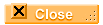Answer 2 8.1.2   Approximately [-2.12, 4.12] x [-1, 2].Lesson 2 Answer 1 8.2.1 The function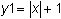is not differentiable at x = 0 because it is not locally linear there. The function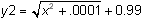is differentiable at x = 0 because it is locally linear there.Lesson 3 Answer 1 8.3.1 x = -2: dy/dx = -4; x = -1: dy/dx = -2; x = 0: dy/dx = 0; x = 1: dy/dx = 2; x = 2: dy/dx = 4Answer 2 8.3.2 The derivative is 2.4, which represents the slope of the tangent line to f(x) = x2 at x = 1.2.Answer 3 8.3.2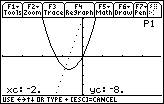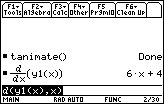The derivative of f(x) = 3x2 + 4x is the function g(x) = 6x + 4.Self Test Answer 1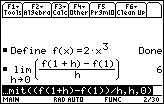Answer 2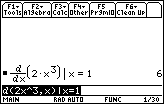Answer 3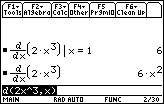Answer 4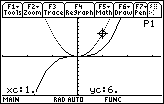Answer 5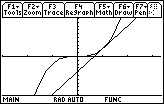The tangent line is y = 6(x – 1) + 2, which is shown with the function in a [–3, 3.32] x [–10, 10] window.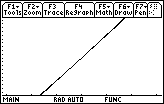The graph is shown after zooming in three times centered on (1, 2) with zoom factors xFact = yFact = 10.©Copyright 2007 All rights reserved. | Trademarks | Privacy Policy | Link Policy